Modern Chemistry
Volume 4, Issue 1, February 2016, Pages: 6-15

Effect of Silver(I) Catalyst on the Oxidation of L-asparagine by Alkaline Hexacyanoferrate(III): A Kinetic and Mechanistic Approach

Ahmed Fawzy1, 2, *, Ishaq Zaafarany1, Ameena Al-Bonayan1, Zakiya Al-Mallah1, Reem Shah1

1Chemistry Department, Faculty of Applied Science, UmmAl-Qura University, Makkah, Saudi Arabia

2Chemistry Department, Faculty of Science, Assiut University, Assiut, Egypt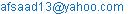(A. Fawzy)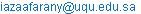(I. Zaafarany)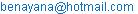(A. Al-Benayan)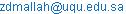(Z. Almallah)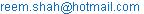(R. Shah)

Ahmed Fawzy, Ishaq Zaafarany, Ameena Al-Bonayan, Zakiya Al-Mallah, Reem Shah. Effect of Silver(I) Catalyst on the Oxidation of L-asparagine by Alkaline Hexacyanoferrate(III): A Kinetic and Mechanistic Approach. Modern Chemistry. Vol. 4, No. 1, 2016, pp. 6-15. doi: 10.11648/j.mc.20160401.12

Abstract: The kinetics of oxidation of L-asparagine (Asn) by hexacyanoferrate(III) (HCF) has been investigated in alkaline medium in the absence and presence of silver(I) catalyst at a constant ionic strength of 0.5 mol dm−3and at 20°C. The progress of both uncatalyzed and silver(I)-catalyzed oxidations was followed spectrophotometrically. Both reactions showed a first order dependence with respect to [HCF], whereas the orders with respect to [Asn] and [OH] were less than unity. The catalyzed reaction exhibited a first order dependence in [AgI]. Increasing both ionic strength and dielectric constant of the reaction medium increased the rate of uncatalyzed reaction and did not affect significantly the rate of catalyzed reaction. Addition of the reaction product, HCF(II) to the reaction mixture had no affect on the rate. Appropriate reaction mechanisms for both uncatalyzed and catalyzed oxidations explaining all of the observed kinetic results has been proposed. The catalyzed reaction has been shown to proceed via formation of a silver(I)-asparagine intermediate complex, which reacted with the oxidant by an inner-sphere mechanism leading to decomposition of the complex in the rate-determining step to yield the final oxidation products which were identified as α-formyl acetamide, ammonia, and carbon dioxide. The rate law expressions associated with the reaction mechanisms were derived.

Keywords: L-asparagine, Hexacyanoferrate(III), Silver(I), Kinetics, Mechanism, Oxidation

1. Introduction

Oxidation reactions are of fundamental importance in nature and are regarded as key transformations in organic synthesis. Also, studying of amino acids is one of the most exciting fields of organic chemistry. Therefore, numerous kinetic investigations of the oxidation of amino acids have been carried out using various oxidants under different experimental conditions , because of their biological significance, selectivity towards the oxidants and the importance of understanding the mechanism of such biological redox reactions. In many cases [3-8, 12-19], it was reported that amino acids undergo oxidative decarboxylation and deamination. L-Asparagine (Asn) is one of the amino acids that occur in relatively high concentrations in plant tissues. It finds extensive applications in the production of pharmaceuticals and medicine, and as a reducing agent in chemical and biochemical systems. The kinetics of oxidation of L-asparagine has been previously studied [1-8]. The rate of oxidation of L-asparagine was found to depend on the oxidant nature and pH of the medium.

Hexacyanoferrate(III) (HCF) is an efficient one-electron oxidant that has high stability, water solubility and moderate reduction potential of 0.45 V during reduction to hexacyanoferrate(II), a stable product . The chemistry of hexacyanoferrate(III) in alkaline medium, particularly its oxidative capacity in oxidation of organic compounds  is well understood. The kinetics of oxidation of some amino acids by hexacyanoferrate(III) in alkaline media have been studied earlier [18-23]. It was observed that such reactions proceed very slowly in the absence of a catalyst, but they proceed more rapidly with the use of some metal ion catalysts. Kinetic studies on the oxidation reactions of amino acids catalyzed by different metal ions are an important field of chemistry because of the role played by metals in biological systems [5-9, 15-24].

A literature survey revealed that no work, however, has been reported on the oxidation of L-asparagine by alkaline hexacyanoferrate(III) either in the absence or presence of any catalyst. The present study deals with the title reactions in order to examine the selectivity of L-asparagine towards hexacyanoferrate(III) in an alkaline medium, to determine the catalytic activity of the silver(I) catalyst, to understand the kinetically active species of the reactants, to identify the reaction products and finally to propose appropriate reaction mechanisms.

2. Experimental

2.1. Materials

All chemicals employed in the present work were of reagent grade, and their solutions were prepared by dissolving the requisite amounts of the samples in doubly distilled water. A stock solution of L-asparagine was freshly prepared by dissolving the amino acid sample (E. Merck, UK) in bi-distilled water. A fresh solution of hexacyanoferrate(III) was prepared by dissolving potassium hexacyanoferrate(III) (BDH) in water, and its concentration was ascertained spectrophotometrically. Hexacyanoferrate(II) solution was prepared by dissolving potassium hexacyanoferrate(II) (S. D. Fine Chem.) in water and standardizing with cerium(IV) solution . Sodium hydroxide and sodium perchlorate were used to vary the alkalinity and ionic strength of the reactions medium, respectively.

2.2. Kinetic Measurements

All kinetic measurements were performed under pseudo-first order conditions where L-asparagine was present in a large excess over hexacyanoferrate(III). The courses of both uncatalyzed and silver(I)-catalyzed reactions were followed by monitoring the decrease in the absorbance of HCF as a function of time at λ = 421 nm (the absorption maximum of HCF) where the other constituents of the reaction mixtures did not absorb significantly at this wavelength. The absorbance measurements were made in a thermostatted Shimadzu UV-VIS-NIR-3600 double-beam spectrophoto-meter.

The oxidation of L-asparagine by hexacyanoferrate(III) in alkaline medium was found to proceed with a slow rate in the absence of silver(I) catalyst. The catalysed reaction is understood to occur in parallel path, with contributions from both the uncatalyzed and catalyzed reactions. Thus, the total rate constant (kT) is equal to sum of the rate constants of the uncatalyzed (kU) and catalyzed (kC) reactions, such that: kC = kT − kU. First order plots of ln(absorbance) versus time were found to be straight lines up to at least about 75% completion of the reactions, and the observed first order rate constants (kU and kC) were calculated as the slopes of such plots. Average values of at least two independent measurements of the rate constants were taken for the analysis. The rate constants were reproducible to within 4%.

The orders with respect to the reactants for both the uncatalyzed and catalyzed reactions were determined from the slopes of the log kU and log kC versus log(concentration) plots by varying the concentrations of substrate, alkali and catalyst, in turn, while keeping other conditions constant.

3. Results

3.1. Stoichiometry and Product Identification

Reaction mixtures containing different initial concentrations of the reactants at [OH-] = 0.4 and I = 0.5 mol dm-3 were equilibrated away from light. The unconsumed concentration of HCF was estimated periodically by spectrophotometric technique. A stoichiometric ratio of ([HCF] / [Asn]) was found to be 2.0 ± 0.11 mol. This result confirms the following stoichiometric equation: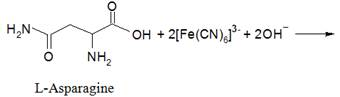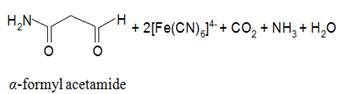The product aldehyde, α-formyl acetamide, was tested by 2,4-dinitrophenylhydrazine . The byproducts were identified as ammonia and carbon dioxide by Nessler’s reagent  and lime water, respectively.

3.2. Spectral Changes

Spectral scans during the oxidation of L-asparagine by hexacyanoferrate(III) in alkaline medium are shown in Figure 1a and b, in the absence and presence of silver(I) catalyst, respectively. In both cases, the scanned spectra indicate gradual disappearance of the HCF(III) band with time as a result of its reduction to HCF(II).

3.3. Effect of [HCF] on the Oxidation Rates

The concentration of the hexacyanoferrate(III) oxidant was varied in the range 3.0–11.0 × 10−4mol dm3, while other variables such as the concentrations of the reductant, silver(I) catalyst and sodium perchlorate, and the pH and temperature were also kept constant. It is evident that the increase in the oxidant concentration did not alter the oxidation rates of L-asparagine (Table 1). This indicates that the oxidation rates were independent of oxidant concentration, and confirms that the order of reactions with respect to the oxidant was unity.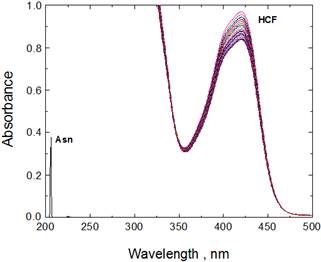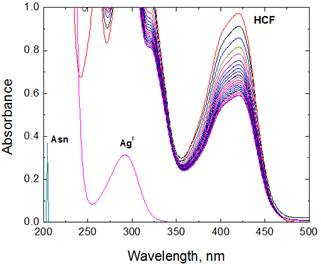(a)                                                                           (b)

Figure 1. Spectral changes during: (a) uncatalyzed, and (b) silver(I)-catalyzed oxidations of L-asparagine by hexacyanoferrate(III) in alkaline medium. [Asn] = 1.5 x 10-2, [HCF] = 7.0 x 104, [OH] = 0.4 and I = 0.5 mol dm−3 at 20°C. [AgI] = 6.0 x 10−5mol dm−3.

Table 1. Effect of variation of [HCF], [Asn], [OH], [AgI] and ionic strength, I, on the observed first order rate constants in the uncatalyzed and silver(I)-catalyzed oxidations of L-asparagine by hexacyanoferrate(III) in alkaline medium at 20°C.

 104 [HCF] (mol dm−3) 102 [Asn] (mol dm−3) [OH−] (mol dm−3) 105 [AgI] (mol dm−3) I (mol dm−3) 103kU (s−1) 103kC (s−1) 3.0 1.5 0.4 6.0 0.5 8.1 38.6 5.0 1.5 0.4 6.0 0.5 7.7 37.2 7.0 1.5 0.4 6.0 0.5 8.2 37.6 9.0 1.5 0.4 6.0 0.5 8.8 38.0 11.0 1.5 0.4 6.0 0.5 7.8 36.9 7.0 0.5 0.4 6.0 0.5 4.2 18.1 7.0 1.0 0.4 6.0 0.5 6.5 27.9 7.0 1.5 0.4 6.0 0.5 8.2 37.6 7.0 2.0 0.4 6.0 0.5 10.5 45.3 7.0 3.0 0.4 6.0 0.5 12.2 53.0 7.0 1.5 0.1 6.0 0.5 3.2 13.9 7.0 1.5 0.2 6.0 0.5 4.9 22.1 7.0 1.5 0.3 6.0 0.5 6.6 30.4 7.0 1.5 0.4 6.0 0.5 8.2 37.6 7.0 1.5 0.5 6.0 0.5 10.3 43.3 7.0 1.5 0.4 2.0 0.5 8.2 11.2 7.0 1.5 0.4 4.0 0.5 8.2 24.8 7.0 1.5 0.4 6.0 0.5 8.2 37.6 7.0 1.5 0.4 8.0 0.5 8.2 48.3 7.0 1.5 0.4 10.0 0.5 8.2 58.2 7.0 1.5 0.4 6.0 0.5 8.2 37.6 7.0 1.5 0.4 6.0 0.6 9.7 38.4 7.0 1.5 0.4 6.0 0.7 10.9 36.9 7.0 1.5 0.4 6.0 0.8 11.9 35.8 7.0 1.5 0.4 6.0 0.9 13.3 39.0

Experimental error ± 4%

3.4. Effect of [Asn] on the Oxidation Rates

The observed rate constants (kU and kC) were determined at different initial concentrations of the L-asparagine, while maintaining other species at fixed concentrations. The plots of the observed rate constants versus [Asn] at constant pH were linear with positive intercepts (Figure 2). These observations confirm that the dependences with respect to the amino acid were fractional-first orders for both the uncatalyzed and catalyzed reactions.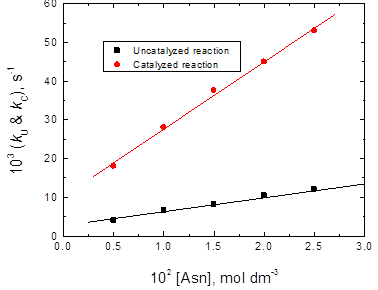Figure 2. Plots of kU and kC versus [Asn] in the uncatalyzed and silver(I)-catalyzed oxidations of L-asparagine by hexacyanoferrate(III) in alkaline medium. [HCF] = 7.0 × 10−4, [OH] = 0.4 and I = 0.5 mol dm−3 at 20°C. [AgI] = 6.0 × 10−5mol dm−3.

3.5. Effect of [OH-] on the Oxidation Rates

The reaction rates were measured at constant [Asn], [HCF], [AgI] (for the catalyzed reaction), ionic strength and temperature, but with varying [OH] (0.1– 0.5 mol dm−3). The rates of the reactions increased with increasing [OH]. Plots of kU and kC versus [OH] were linear with positive intercepts, as shown in Figure 3, confirming fractional-first order dependences with respect to [OH].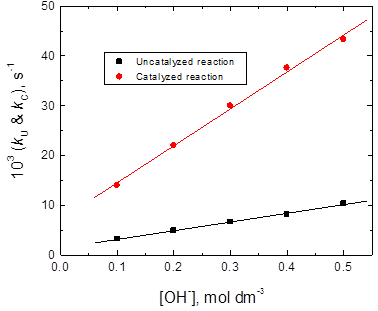Figure 3. Plots of kU and kC versus [OH] in the uncatalyzed and silver(I)-catalyzed oxidations of L-asparagine by hexacyanoferrate(III) in alkaline medium. [HCF] = 7.0 × 10−4, [Asn] = 1.5 x 10-2 and I = 0.5 mol dm−3 at 20°C. [AgI] = 6.0 × 10−5mol dm−3.

3.6. Effect of [AgI] on the Oxidation Rate

The silver(I) catalyst concentration was varied from 2.0 × 10−5 to 1.0 × 10−4mol dm−3 at constant [Asn], [HCF], [OH], ionic strength and temperature. The reaction rate was found to increase linearly with increasing [AgI] (Table 1) and the order with respect to silver(I) catalyst was unity as obtained from the slope of the log kC versus log[AgI] plot (Figure 4).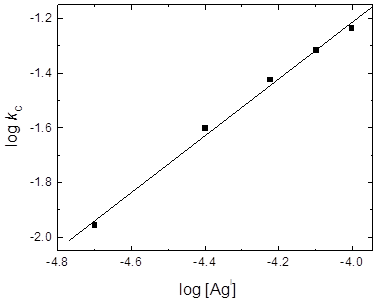Figure 4. Plot of log kC versus log [AgI] in the silver(I)-catalyzed oxidation of L-asparagine by hexacyanoferrate(III) in alkaline medium. [Trp] = 1.5 x 10-2, [HCF] = 7.0 × 10−4, [OH] = 0.4 and I = 0.5 mol dm−3 at 20°C.

3.7. Effect of Ionic Strength and Dielectric Constant on the Oxidation Rates

In order to investigate the effect of ionic strength on both the uncatalyzed and catalyzd reactions, the reactions were studied at several initial concentrations of sodium perchlorate with constant concentrations of the reactants, and at constant pH and temperature. The results presented in Table 1show that increasing ionic strength increased the rate of uncatalyzed reaction and did not affect significantly the rate of catalyzed reaction, and the Debye-Hückel plot in case of the uncatalyzed reaction was found to be linear with a positive slope as shown in Figure 5a.

The effect of dielectric constant, D, was studied by varying the t-butyl alcohol – water content in the reaction mixtures at 20°C. The rate constant of the uncatalyzed reaction was found to decrease with decreasing dielectric constant of the solvent mixture, i.e. increasing t-butyl alcohol content, whereas that of the catalyzed reaction did not significantly affected. The plot of log kU versus 1/D was linear with a negative slope (Figure 5b).

3.8. Effect of Initially Added Product

The effect of addition of the product hexacyanoferrate(II) was also studied in the concentration range 3.0 –12.0 × 10−4 mol dm−3 at fixed concentrations of the oxidant, reductant, alkali and catalyst. HCF(II) had no significant effect on the rate of reaction.

3.9. Polymerization Test

Known amounts of acrylonitrile scavenger were added to reaction mixtures, which were kept for 4 h in an inert atmosphere. On dilution of the mixtures with methanol, white precipitates were formed, thus confirming the presence of free radicals intervention in these reactions. When these experiments were repeated in the absence of L-asparagine under otherwise similar conditions, the tests were negative. This indicates that the reactions proceeded via free radical pathways.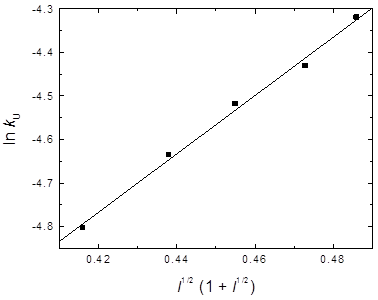(a)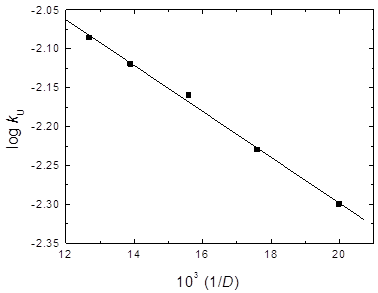(b)

Figure 5. Effect of (a) ionic strength, I, and (b) dielectric constant, D, of the medium on the uncatalyzed oxidation of L-asparagine by hexacyanoferrate(III) in alkaline medium. [Asn] = 1.5 x 10-2, [HCF] = 7.0 × 10−4 and [OH] = 0.4 mol dm−3 at 20°C.

4. Discussion

4.1. Mechanism of the Uncatalyzed Oxidation Reaction

Hexacyanoferrate(III) oxidation of L-asparagine in alkaline medium was found to occur at a slow rate in the absence of the silver(I) catalyst. The reaction had a stoichiometry of 2:1, i.e. two moles of hexacyanoferrate(III) reacted with one mole of L-asparagine. The reaction exhibited first order dependence with respect to [HCF] and less than unit order with respect to [Asn]. The rate of HCF reduction increased with increasing [OH] with a fractional-first order dependence, suggesting deprotonation of L-asparagine by the alkali prior to the rate-determining step that forms a more reactive species of the reductant . The rate was not affected by addition of HCF(II), indicating that the possibility of a fast equilibrium with the product preceding the rate-determining step can be ruled out. Therefore, the rate-determining step should be irreversible, as is generally the case for one-electron oxidants , and the oxidation takes place through generation of a free radical, as observed experimentally. In addition, the rate of reaction increased with the increase in the ionic strength and dielectric constant of the medium, suggesting that the reaction occurs between two similarly charged ions [37, 38].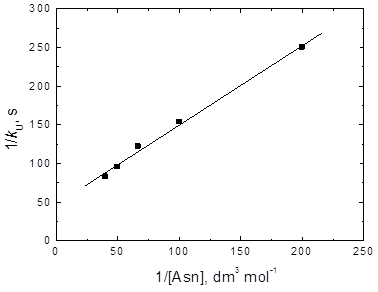Figure 6. Verification of equations (10) in the uncatalyzed oxidation of L-asparagine by hexacyanoferrate(III) in alkaline medium. [HCF] = 7.0 × 10−4, [OH-] = 0.4 and I = 0.5 mol dm−3 at 20°C.

On the other hand, the less than unit order in [Asn] may be due to formation of a complex (C1) between the HCF species and the deprotonated L-asparagine species prior to the rate-determining step. Complex formation was also proved kinetically by the non-zero intercept of the plot 1/kU versus 1/[Asn] (Figure 6) in favor of possible formation of an intermediate complex between the oxidant and substrate . The formed complex (C1) is slowly decomposed in the rate-determining step to give rise to the initial oxidation products as the substrate intermediate radical (Asn.) and HCF(II). This is followed by decarboxylation of L-asparagine free radical, forming a new radical intermediate (X.). This reacts with another HCF species in a subsequent fast step to give rise to the final oxidation products, as illustrated in the following sequence: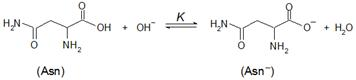(1)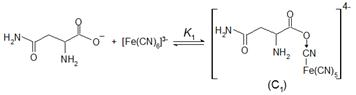(2)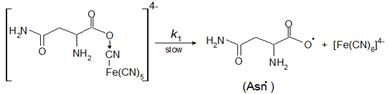(3)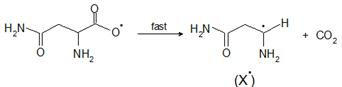(4)(5)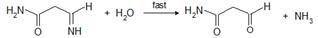(6)

The suggested mechanism leads to the following rate law expression (see Appendix A),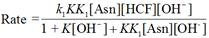(7)

The above rate law is consistent with all observed orders with respect to the different species.

Under the pseudo-first order condition, the rate-law can be expressed by Eq. (8),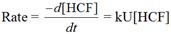(8)

Comparing Eqs. (7) and (8), the following relationship is obtained,(9)

Equation (9) can be rearranged to the following forms, which are suitable for verification,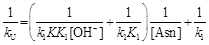(10)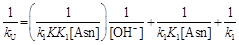(11)

According to Eq. (10), a plot of 1/kU versus 1/[Asn] at constant [OH] should be linear with a positive intercept. This is verified in Figure 6. The intercept corresponds to 1/k1, from which the value of k1 of 21.3 × 10−3 s−1 at 20°C is determined. Similarly, on the basis of Eq. (11), the plot of 1/kU versus 1/[OH] at a constant substrate concentration (Figure 7) yields a straight line with slope and intercept equal to 1/k1KK1[Asn] and 1/k1K1[Asn], respectively. Now, with the help of the slope and intercept of such plot, the calculated values of K and K1 at 20°C were 0.14 and 783.4 dm3 mol−1, respectively.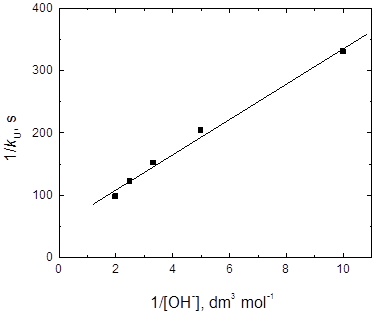Figure 7. Verification of equations (11) in the uncatalyzed oxidation of L-asparagine by hexacyanoferrate(III) in alkaline medium. [HCF] = 7.0 × 10−4, [Asn] = 1.5 x 10-2 and I = 0.5 mol dm−3 at 20°C.

4.2. Mechanism of the Silver(I)-Catalyzed Oxidation Reaction

The reaction between HCF and L-asparagine in alkaline medium in the presence of small amounts of silver(I) catalyst is similar to the uncatalyzed reaction with respect to the stoichiometry and the reaction orders and it different with respect to the influence of both ionic strength and dielectric constant of the medium where the latter did not affect the reaction rate. The reaction was first order with respect to [AgI]. The less than unit order with respect to [Asn] may be as a results of a complex formation between the L-asparagine substrate and silver(I) catalyst in a pre-equilibrium step before the reaction with the oxidant. The formation of the complex was proved kinetically by the non-zero intercept of the [AgI]/kC versus 1/[Asn] plot (Figure 8). Such complexes between L-asparagine and silver(I) catalyst have been reported in earlier studies [6,8].

In view of the abovementioned aspects, deprotonated L-asparagine is suggested to combine with a AgI to form a complex (C2) prior to the rate-determining step. The oxidant HCF then attacks this complex in the rate-determining step to form L-asparagine free radical and HCF(II), with regeneration of the catalyst AgI. This is subsequently followed by fast steps that give rise to the final oxidation products, as shown in the following sequence: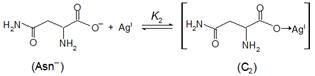(12)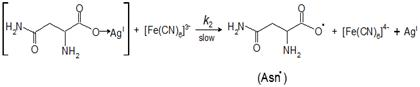(13)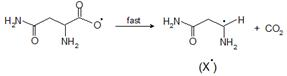(14)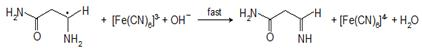(15)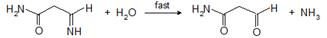(16)

An alternative reaction mechanism [18, 40] for metal ion-catalyzed oxidation may be proposed. It involves the formation of an intermediate complex (C2) between the metal ion catalyst and the amino acid that on further interaction with the oxidant in the rate-determining step yields another complex (C3) of a higher valence metal ion and the reduced form of the oxidant. Such a complex is rapidly decomposed to give rise to the intermediate radical with regeneration of the catalyst, subsequently followed by fast steps to yield the final oxidation products, as illustrated in the following equations: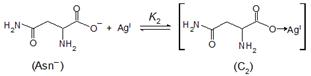(17)(18)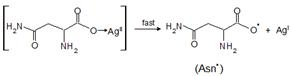(19)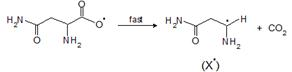(20)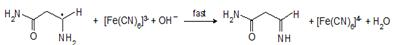(21)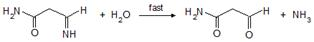(22)

The suggested mechanism leads to the following rate law expression,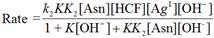(23)

Also, the above rate law is consistent with all observed orders with respect to different species.

Under a pseudo-first order condition, the rate-law can be expressed by Eq. (23),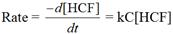(24)

Comparing Eqs. (23) and (24), the following relationship is obtained,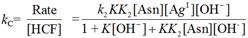(25)

Equation (25) can be rearranged to the following forms, which are suitable for verification,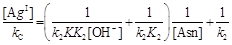(26)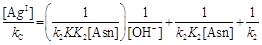(27)

Equations (26) and (27) require that plots of [AgI]/kC versus 1/[Asn] at constant [OH] and [AgI]/kC versus 1/[OH] at constant [Asn], respectively, to be linear with positive intercepts on the [AgI]/kC axes. These requirements are verified in Figures 8 and 9, respectively. Similarly to the uncatalyzed reaction, values of k2, K and K2at 20°C are calculated from the slopes and intercepts of the aforementioned plots to be 1.5 x 103 mol dm−3 s−1, 0.23 dm3 mol−1and 406.1 dm3 mol−1, respectively.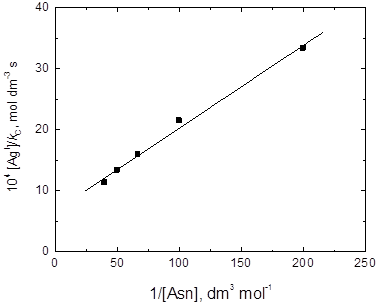Figure 8. Verification of equations (26) in the silver(I)-catalyzed oxidation of L-asparagine by hexacyanoferrate(III) in alkaline medium. [HCF] = 7.0 × 10−4, [OH-] = 0.4, [AgI] = 6.0 x 10-5 and I = 0.5 mol dm−3 at 20°C.

5. Conclusions

A comparative study of uncatalyzed and silver(II)-catalyzed oxidation of L-asparagine by hexacyanoferrate(III) in alkaline medium was performed. The rate of silver(II)-catalyzed reaction are more than four-fold faster than that of the uncatalyzed reaction. The overall sequences described here are consistent with all experimental findings.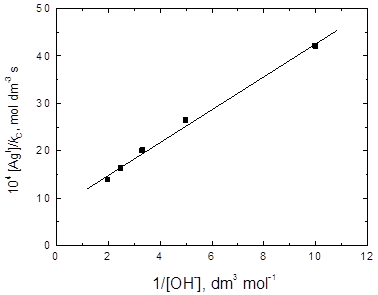Figure 9. Verification of equations (27) in the silver(I)-catalyzed oxidation of L-asparagine by hexacyanoferrate(III) in alkaline medium. [HCF] = 7.0 × 10−4, [Asn] = 1.5 x 10-2, [AgI] = 6.0 x 10-5 and I = 0.5 mol dm−3 at 20°C.

Appendix A:

Derivation of Rate Law for Uncatalysed Reaction

According to the proposed mechanistic Scheme 1,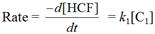(A1)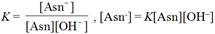(A2)

and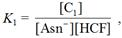[C1] = K1[Asn-][HCF] = KK1[Asn][HCF][OH]     (A3)

Substituting Eq. (A3) into Eq. (A1) leads to,

Rate = k1KK1[Asn][HCF][OH]            (A4)

The total concentration of Asn is given by,

[Asn]T = [Asn]F + [Asn] + [C1]            (A5)

where ‘T’ and ‘F’ stand for total and free concentrations.

Substituting Eqs. (A2) and (A3) into Eq. (A5), and rearrangement gives,

[Asn]T = [Asn]F + K[Asn][OH] + KK1[Asn][HCF][OH]   (A6)

[Asn]T = [Asn]F (1+ K[OH] + KK1[HCF][OH])     (A7)

Therefore,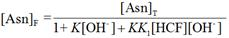(A8)

In view of the low [HCF], the third denominator term, KK1[HCF][OH], in the above equation can be neglected. Therefore,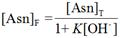(A9)

Also,

[HCF]T = [HCF]F + [C1]              (A10)

Substituting Eq. (A3) into Eq. (A10) gives,

[HCF]T = [HCF]F(1 + KK1[Asn][OH])       (A11)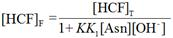(A12)

In view of the concentration of [OH],

[OH-]F = [OH]T                 (A13)

Substituting Eqs. (A9), (A12) and (A13) into Eq. (A4) (and omitting ‘T’ and ‘F’ subscripts) gives,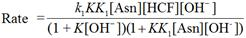(A14)

Under pseudo-first order condition, the rate-law can be expressed by Eq. (A15),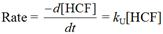(A15)

Comparing Eqs. (A14) and (A15), the following relationship is obtained,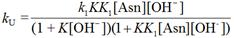(A16)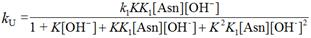(A17)

In view of the low concentration of Asn used, the term K2K1[Asn][OH] in the fourth denominator of Eq. (A17) is negligibly small compared to unity. Therefore, Eq. (A17) can be written as,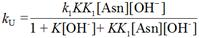(A18)

References

1. Senagar SKS, Yadav BS (1988) Kinetics and mechanism of copper(II)–catalyzed oxidation of asparagine by sodium N-chloro-p-toluene sulphonamide in alkaline media, J. Indian Chem. Soc. 65: 88–90.
2. Sanjeevagowda TP, Mahantesh AA, Abdulazizkhan LH (2008) Oxidative deamination and decarboxylation of L-asparagine by the aqueous alkaline diperiodato-nickelate(IV) complex, J. Solution Chem. 37: 1795–180.
3. Fawzy A (2015) Kinetics and mechanistic approach to the oxidative behavior of biological anticancer platinum(IV) complex towards L-asparagine in acid medium and the effect of copper(II) catalyst Int. J. Chem. Kinet. 47: 1-12.
4. Khalid MAA (2007) Oxidative kinetics of amino acids by peroxydisulfate: Effect of dielectric constant, Arabian J. Sci. Eng. 33: 199-210.
5. Fawzy A, Zaafarany IA (2015) Mechanistic investigation of copper(II)-catalyzed oxidation of L-asparagine by hexachloroplatinate(IV) in aqueous alkaline medium: a kinetic approach.J. Multidisc. Eng. Sci. Technol.,2: 1038-1045.
6. Asghar BH, Altass HM, Fawzy A (2016) Silver(I)-catalysis of oxidative deamination and decarboxylation of L-asparagine and L-histidine by platinum(IV) in perchloricacid solutions: acomparative kinetics study. J. Env. Chem. Eng.4:617-623.
7. Fawzy A, Ashour SS, Musleh MA (2014) Base-catalyzed oxidation of L-asparagine by alkaline permanganate and the effect of alkali-metal ion catalysts: Kinetics and mechanistic approach, React. Kinet.Mech. Catal. 111: 443-460.
8. Asghar BH, Altass HM, Fawzy A (2015) Transition metal ions-catalyzed oxidation of L-asparagine by platinum(IV) in acid medium: a kinetic and mechanistic study. Transition Met. Chem. 40: 587–594.
9. Anweting IB, Iyun JF, Idris SO (2012) Kinetics and mechanistic approach to the oxidation of L-tryptophan by permanganate ion in aqueous acidic medium. Adv. Appl. Sci. Res. 3: 3401-3409.
10. Sharma VK, Sharma K, Tiwari PS, Khare D (2011) Mechanism of quinquevalent vanadium oxidation of L-typtophan in sulphuric acid medium. Int. J. Pharm. Life Sci. 2: 1223-1225.
11. Shetti NP, Hosamani RR, Nandibewoor ST (2009) Kinetic and mechanistic investigations on oxidation of L-tryptophan by diperiodatocuprate (III) in aqueous alkaline medium. Open Catal. J. 2: 130-139.
12. Fawzy A, Ashour SS, Musleh MA (2014) Kinetics and mechanism of oxidation of L-histidine by permanganate ions in sulfuric acid medium, Int. J. Chem. Kinet. 46: 370-381.
13. Fawzy A, Althagafi I (2016) Kinetics and mechanism of silver(I)-catalyzed oxidation of tryptophan by platinum(IV) in perchlorate solutions, Am. J. Chem. Eng. In press.
14. Fawzy A, Ashour SS, Musleh MA, Hassan RM, Asghar BH (2015) Kinetics and mechanistic approach to the chromic acid oxidation of L-tryptophan with a spectral detection of chromium(III) product, J. Saudi Chem. Soc. in press.
15. Fawzy A (2014) Influence of Copper(II) Catalyst on the Oxidation of L-Histidine by Platinum(IV) in Alkaline Medium: A Kinetic and Mechanistic Study. Transition Met. Chem. 39: 567-576.
16. Fawzy A, Asghar BH (2015) Kinetics and mechanism of uncatalyzed and silver(I)-catalyzed oxidation of L-histidine by hexachloroplatinate(IV) in acid medium, Transition Met. Chem. 40: 287-295.
17. Fawzy A, Zaafarany IA (2015) Kinetic and mechanistic investigation on the zirconium(IV)-catalyzed oxidation of L-histidine by hexachloroplatinate(IV) in acid medium. Chem. Sci. Rev. Lett., 4: 608-618.
18. Asghar BH, Altass HM, Fawzy A (2015) Copper(II) catalysis for oxidation of L-tryptophan by hexacyanoferrate(III) in alkaline medium: a kinetic and mechanistic approach, J. Saudi.Chem. Soc. in press.
19. Fawzy A (2015) Palladium(II)-catalyzed oxidation of l-tryptophan by hexacyanoferrate(III) in perchloric acid medium: a kinetic and mechanistic approach. J. Chem. Sci.In press.
20. Sharanabasamma K, Angadi MA, Salunke MS, Tuwar SM (2009) Osmium(VIII) catalysed oxidative cleavage of pyrrolidine ring in L-proline by hexacyanoferrate(III) in alkaline media. Ind. Eng. Chem. Res. 48: 10381–10386.
21. Goel A, Sharma S (2010)Mechanistic study of the oxidation of L-phenylalanine by hexacyanoferrate(III) catalyzed by iridium(III) in aqueous alkaline medium, Transition Met. Chem. 35: 549-554.
22. Devra V, Yadav MB (2012) Kinetics and mechanism of osmium(VIII) catalyzed oxidation of valine by hexacyanoferrate in alkaline medium, Rassian J. Chem. 5: 67-73.
23. Upadhyay SK, MC agrawal (1977) Kinetics of Os(VIII)-catalyzed alkaline hexacyanoferrate(III) oxidation of some α-amino acids in presence of excess of ferricyanide, Ind. J. Chem., 15A:709-712;
24. Kelson EP, Phengsy PP (2000) Kinetic study of 2-propanol and benzyl alcohol oxidation by alkaline hexacyanoferrate(III) catalysed by a terpyridyl ruthenium complex. Int. J. Chem. Kinet. 32: 760–770.
25. Vovk AI, Muraveva IV, Kukhar VP, Baklan VF (2000) Kinetics of oxidation of vitamin B1 and its Oacyl analogs with ferricyanide. A mechanistic model of thiamin-binding protein. Russ. J. Gen. Chem. 70: 1108–1112.
26. Speakman PT, Waters WA (1955) Kinetic features of the oxidation of aldehydes, ketones and nitroparaffins with alkaline ferricyanide. J. Chem. Soc. 40–50.
27. Jose TP, Nandibewoor ST, Tuwar SM (2006) Kinetics and mechanism of oxidation of vanillin by hexacyanoferrate(III) in aqueous alkaline medium. J. Solution Chem. 35: 51–62.
28. Singh VN, Singh MP, Saxena BBL (1970) Kinetics and mechanism of alkaline ferricyanide oxidation of acetone and ethyl methyl ketone. Indian J. Chem. 8: 529–532.
29. Leal JM, Garcia B, Domingo PL (1998) Outer-sphere hexacyanoferrate(III) oxidation of organic substrates. Coord. Chem. Rev. 173: 79–131.
30. Jose TP, Angadi MA, Salunke MS, Tuwar SM (2008) Oxidative study of gabapentin by alkaline hexacyanoferrate(III) in room temperature in presence of catalytic amount of Ru(III). A mechanistic approach. J. Mol. Struct. 892: 121–124.
31. Farokhi S A, Nandibewoor S T (2003) Kinetic, mechanistic and spectral studies for the oxidation of sulfanilic acid by alkaline hexacyanoferrate(III). Tetrahedron 59: 7595-7601.
32. Jeffery G H, Bassett J, Mendham J, Denney RC (1996) Text Book of Quantitative Chemical Analysis, 5th ed.; ELBS Longman: Essex, pp.384.
33. Feigl F (1975) Spot Tests in Organic Analysis, 195 pp. Elsevier, New York.
34. Vogel AI (1973) Text Book of Practical Organic Chemistry Including Quantitative Organic Analysis, 3rd ed., 332 pp. ELBS, Longman.
35. Chang R (1981) Physical Chemistry with applications to biological systems, Macmillan, New York, NY, USA.
36. Leal J M, Domingo PL, Garcla B, Ibeas S (1993) Alkali metal ion catalysis of the oxidation of L- ascorbic acid by hexacyanoferrate(III) in strongly acidic media. J. Chem. Soc. Faraday Trans. 89: 3571–3577.
37. Frost A A, Person R G (1973) Kinetics and mechanism,147pp. Wiley Eastern, New Delhi.
38. Amis E S (1966) Solvent Effect on Reaction Rates and Mechanism, pp.28, Academic Press, NewYork.
39. Michaelis L, Menten M L (1913) The kinetics of invertase action. Biochem. Z.49: 333–369.
40. Yadav M B, Derva V, Rani A (2009) Kinetics and mechanism of uncatalyzed and silver(I) catalyzed oxidation of lysine by cerium(IV) in acid perchlorate medium. J. Indian Chem. Soc. 86: 600-604.

 Contents 1. 2. 2.1. 2.2. 3. 3.1. 3.2. 3.3. 3.4. 3.5. 3.6. 3.7. 3.8. 3.9. 4. 4.1. 4.2. 5.
Article ToolsAbstractPDF(1044K)